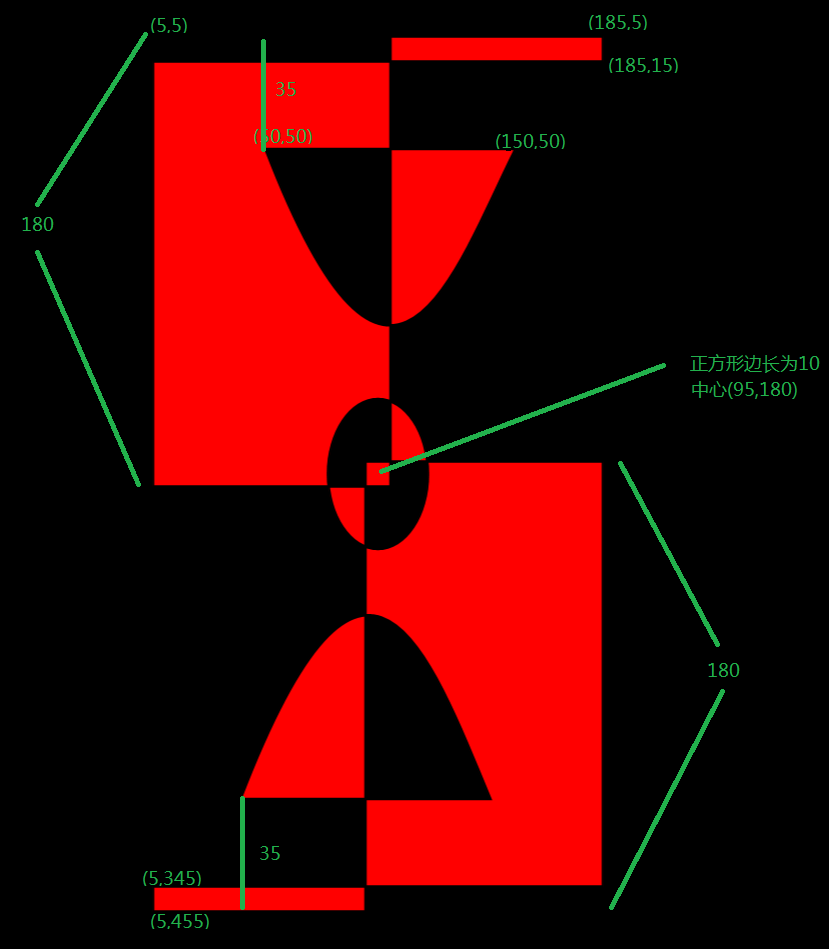# 绘制图形

## Rectangle

``````<Rectangle Fill="Yellow" Width="300" Height="200" Stroke="Blue"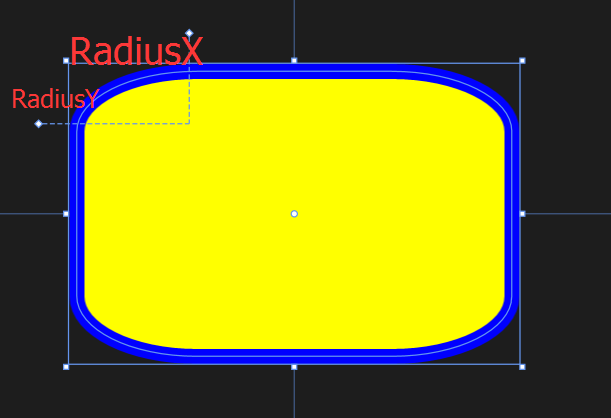## Ellipse

``<Ellipse Fill="Blue" Height="200" Width="350"/>``

## Polygon

Polygon 则显得比较自由，只需要定义出各个顶点，它就会将这些点连接起来。那么我们可能会有疑问，需不需要确定图形的起始点和终点呢？答案是不用的，因为 Polygon 会自动将终点和起始点连接起来（它会假设图形是闭合的）。

``<Polygon Fill="Green" Points="0,0,100,0,100,100,0,100  "/>``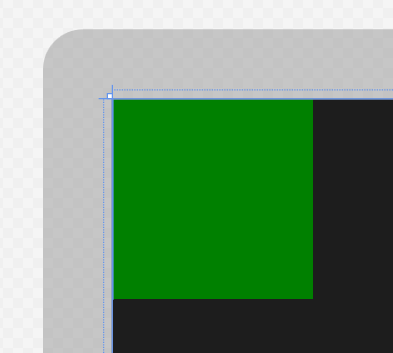## Line

Line 的使用也比较简单，但有一点要注意，必须设置好 Stroke 和 StrokeThickness 的属性值，否则 Line 就不会显示出来。原因很简单，因为它是直线。

``<Line Stroke="Red" StrokeThickness="10" X1="100" Y1="0" Y2="400" X2="400"/>``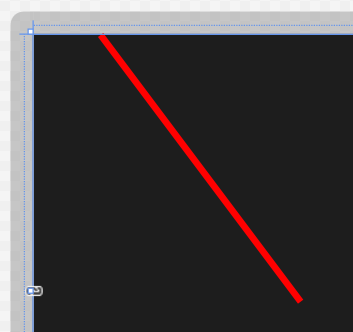## Path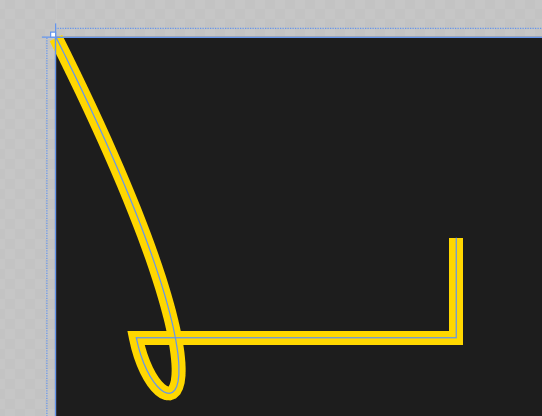``````<Path Stroke="Gold"  StrokeThickness="7"
Data="M 0,0 C 100,200 50,200 40,150 H 200 V 100 "/>``````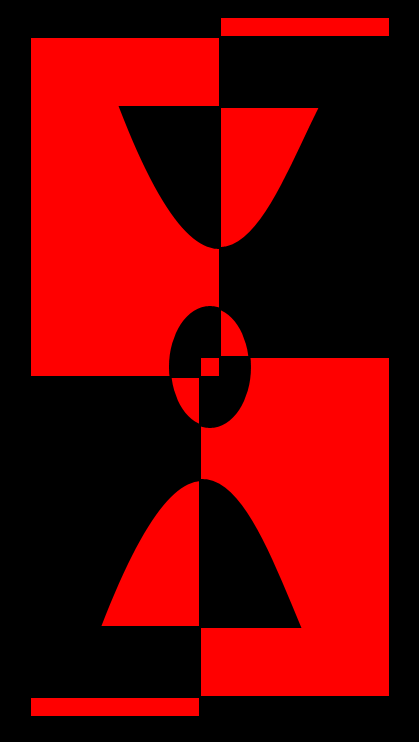``````  <Path Stroke="Black" StrokeThickness="1" Fill="red">
<Path.Data>
<GeometryGroup>
<RectangleGeometry Rect="5,5 180,10" />
<RectangleGeometry Rect="5,5 95,180" />
<RectangleGeometry Rect="90,175 95,180"/>
<RectangleGeometry Rect="5,345 180,10" />
<EllipseGeometry
<PathGeometry>
<PathGeometry.Figures>
<PathFigureCollection>
<PathFigure IsClosed="true" StartPoint="50,50">
<PathFigure.Segments>
<PathSegmentCollection>
<BezierSegment Point1="100,180"
Point2="125,100" Point3="150,50"/>
</PathSegmentCollection>
</PathFigure.Segments>
</PathFigure>
<PathFigure IsClosed="true" StartPoint="40,310">
<PathFigure.Segments>
<PathSegmentCollection>
<BezierSegment Point1="90,180"
Point2="115,250"Point3="140,310"/>
</PathSegmentCollection>
</PathFigure.Segments>
</PathFigure>
</PathFigureCollection>
</PathGeometry.Figures>
</PathGeometry>
</GeometryGroup>
</Path.Data>
</Path>  ``````